# KSEEB Solutions for Class 6 Maths Chapter 3 Playing with Numbers Ex 3.7

Students can Download Chapter 3 Playing with Numbers Ex 3.7 Questions and Answers, Notes Pdf, KSEEB Solutions for Class 6 Maths helps you to revise the complete Karnataka State Board Syllabus and score more marks in your examinations.

## Karnataka State Syllabus Class 6 Maths Chapter 3 Playing with Numbers Ex 3.7

Question 1.
Renu purchases two bags of fertiliser of weights 75 kg and 69 kg. Find the maximum value of weight which can measure the weight of the fertiliser exact number of times.
Solution:
Weight of the two bags = 75 kg and 69 kg
Maximum weight = HCF (75, 69)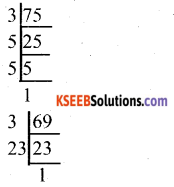75 = 3 × 5 × 3
69 = 3 × 23
HCF = 3
Hence, the maximum value of weight, which can measure the weight of the fertilizer exact number of times, is 3 kgQuestion 2.
Three boys step off together from the same spot. Their steps measure 63 cm, 70 cm and 77 cm respectively. What is the minimum distance each should cover so that all can cover the distance in complete steps?
Solution:
Step measure of 1 Boy = 63 cm
Step measure of 2 Boy = 70 cm
Step measure of 3 Boy = 77 cm
LCM of 63, 70, 77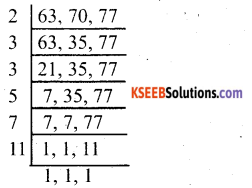LCM = 2 × 3 × 3 × 5 × 7 × 11 = 6930
Hence, the minimum distance each should cover so that all can cover the distance in complete steps is 6930 cm.

Question 3.
The length, breadth and height of a room are 825 cm, 675 cm and 450 cm respectively. Find the longest tape which can measure the three dimensions of the room exactly.
Solution:
Length = 825 cm = 3 × 5 × 5 × 11
Breadth = 675 cm = 3 × 3 × 3 × 5 × 5
Height = 450 cm = 2 × 3 × 3 × 5 × 5
Longest tape = HCF of 825, 675, and 450 = 3 × 5 × 5 = 75 cm
Therefore, the longest tape is 75 cm.Question 4.
Determine the smallest 3-digit number which is exactly divisible by 6, 8 and 12
Solution:
Smallest number = LCM of 6, 8, 12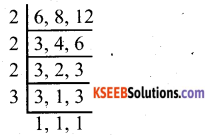LCM = 2 × 2 × 2 × 3 = 24
the smallest 3-digit multiple of 24.
It can be seen that 24 × 4 = 96 and 24 × 5 = 120.
Hence, the smallest 3-digit number which is exactly divisible by 6, 8, and 12 is 120

Question 5.
Determine the greatest 3-digit number exactly divisible by 8, 10 and 12.
Solution:
LCM of 8, 10, and 12
2, 8,10,12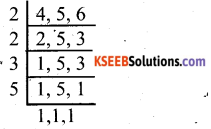LCM = 2 × 2 × 2 × 3 × 5 = 120
We have to and the greatest 3-digit multiple of 120.
It can be seen that 120 × 8 = 960 and 120 × 9 = 1080.
Hence, the greatest 3-digit number exactly divisible by 8, 10, and 12 is 960.Question 6.
The traffic lights at three different road crossings change after every 48 seconds, 72 seconds and 108 seconds respectively. If they change simultaneously at 7 a.m., at what time will they change simultaneously again?
Solution:
Time period after which these lights will change = LCM of 48, 72, 108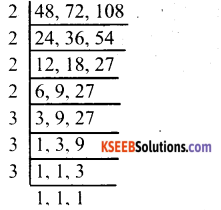LCM = 2 × 2 × 2 × 2 × 3 × 3 × 3 = 432
They will change together after every 432 seconds i.e., 7 min 12 seconds.
Hence, they will change simultaneously at 7 : 07 : 12 am.

Question 7.
Three tankers contain 403 litres, 434 litres and 465 litres of diesel respectively. Find the maximum capacity of a container that can measure the diesel of the three containers exact number of times.
Solution:
Maximum capacity of the required tanker = HCF of 403, 434, 465
403 = 13 × 31
434 = 2 × 7 × 31
465 = 3 × 5 × 31
HCF = 31
A container of capacity 31 l can measure the diesel of 3 containers exact number of times

Question 8.
Find the least number which when divided by 6, 15 and 18 leave remainder 5 in each case.
Solution:
LCM of 6, 15, 18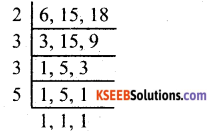LCM = 2 × 3 × 3 × 5 = 90
Required number = 90 + 5 = 95

Question 9.
Find the smallest 4-digit number which is divisible by 18, 24 and 32.
Solution:
LCM of 18, 24, and 32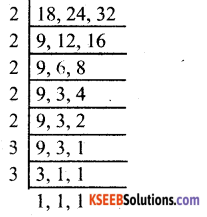LCM = 2 × 2 × 2 × 2 × 2 × 3 × 3 = 288
We have to and the smallest 4-digit multiple of 288.
It can be observed that 288 × 3 = 864 and 288 × 4 = 1152.
Therefore, the smallest 4-digit number which is divisible by 18, 24, and 32 is 1152.Question 10.
Find the LCM of the following numbers:
(a) 9 and 4
(b) 12 and 5
(c) 6 and 5
(d) 15 and 4
Observe a common property in the obtained LCMs. Is LCM the product of two numbers in each case?
Solution:
(a) 9 and 4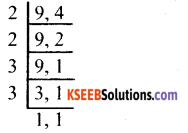LCM = 2 × 2 × 3 × 3 = 36(b) 12 and 5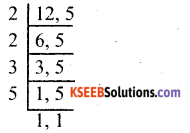LCM = 2 × 2 × 3 × 5 = 60

c) 6 and 5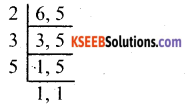LCM = 2 × 3 × 5 = 30

d) 15 and 4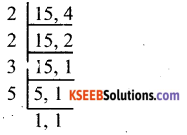LCM = 2 × 2 × 3 × 5 = 60
Yes, it can be observed that in each case, the LCM of the given numbers is the product of these numbers.
When two numbers are co-prime, their LCM is the product of those numbers. Also, in each case, LCM is a multiple of 3.Question 11.
Find the LCM of the following numbers in which one number is the factor of the other.
(a) 5, 20
(b) 6, 18
(c) 12, 48
(d) 9, 45
Solution: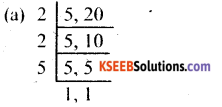LCM = 2 × 2 × 5 = 20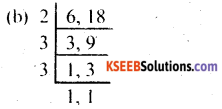LCM = 2 × 3 × 3 = 18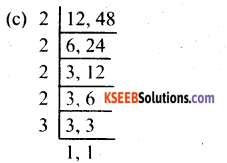LCM = 2 × 2 × 2 × 2 × 3 = 48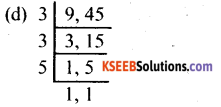LCM = 3 × 3 × 5 = 45
Yes, it can be observed that in each case, the LCM of the given numbers is the larger number. When one number is a factor of the other number, their LCM will be the larger number.